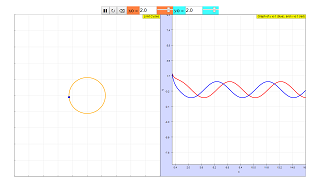Mathematical Model

This simulation illustrates the concept of a limit cycle by using the following mathematical model:

dx/dt = y +  [K*x*(1 - x^2 - y^2)]/sqrt(x^2 +  y^2)

dy/dt = -x + [K*y*(1 - x^2 - y^2)]/sqrt(x^2 +  y^2)

The initial conditions are as follows:

x(0) = x0

y(0) = y0

The limit cycle of this function is a circle centered at the origin with radius 1 (the unit circle), which can be expressed in the following statement.

For all x0,y0 (where x0, y0 are non-zero), x^2 + y^2 approaches 1 as t tends to infinity.

Activities The initial value conditions in the XY-plane can be set by using two sliders and fields. Press the "Initialize", then "Play" button to start the simulation. The model trajectory is displayed. Authors Alfonso Urquía and Carla Martín
Dpto. de Informática y Automática
E.T.S. Ingeniería Informática, UNED
Juan del Rosal 16, 28040 Madrid, Spain

### Translations

Code Language Translator Run### Software Requirements

SoftwareRequirements

 Android iOS Windows MacOS with best with Chrome Chrome Chrome Chrome support full-screen? Yes. Chrome/Opera No. Firefox/ Samsung Internet Not yet Yes Yes cannot work on some mobile browser that don't understand JavaScript such as..... cannot work on Internet Explorer 9 and below

### CreditsAlfonso Urquía; Carla Martín; Tan Wei Chiong; Loo Kang Wee

[text]

### For Teachers

This simulation illustrates the concept of a limit cycle by using the following mathematical model:
dx/dt = y + [K*x*(1 - x^2 - y^2)]/sqrt(x^2 + y^2)
dy/dt = -x + [K*y*(1 - x^2 - y^2)]/sqrt(x^2 + y^2)

The initial conditions are as follows:
x(0) = x0
y(0) = y0

The limit cycle of this function is a circle centered at the origin with radius 1 (the unit circle), which can be expressed in the following statement.
For all x0,y0 (where x0, y0 are non-zero), x^2 + y^2 approaches 1 as t tends to infinity.

In essence, for any point on the Cartesian plane, it will eventually approach the limit of the unit circle, no matter where the point is.

The graph on the left illustrates the path of the point, while the graph on the right illustrates the graph of y against t (blue) and x against t (red).

Research

[text]

[text]

[text]